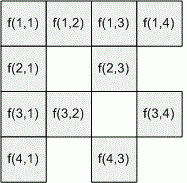favorite We need a little bit of your help to keep things running, click on this banner to learn more
Competitions

# Send a Table

Jimmy have to calculate a function f(x, y) where x and y are both integers in the range [1, n]. When he knows f(x, y), he can easily derive f(k * x, k * y), where k is any integer from it by applying some simple calculations involving f(x, y) and k.

Note that the function f is not symmetric, so f(x, y) can not be derived from f(y, x).

For example if n = 4, he only needs to know the answers for 11 out of the 16 possible input value combinations:The other 5 can be derived from them:

• f(2, 2), f(3, 3) and f(4, 4) from f(1, 1);
• f(2, 4) from f(1, 2);
• f(4, 2) from f(2, 1);

#### Input

Consists of at most 600 lines. Each line contains an integer n (1n50000). The last line contains one zero and should not be processed.

#### Output

For each input value of n print in a separate line the minimum number of function values Jimmy needs to know to compute all n2 values f(x, y).

Time limit 1 second
Memory limit 128 MiB
Input example #1
2
4
5
0

Output example #1
3
11
19